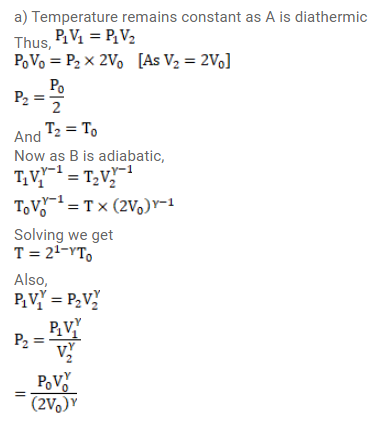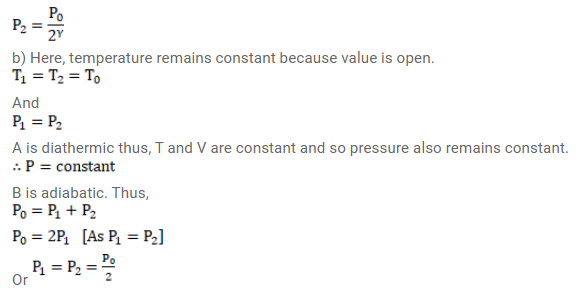# Two vessels A and B of equal V0 are connected by

Question:

Two vessels $A$ and $B$ of equal $V_{0}$ are connected by a narrow tube which can be closed by a valve. The vessels are fitted with pistons which can be moved to change the volumes. Initially, the valve is open and the vessels contain an ideal gas $\left(C_{p} / C_{v}=\gamma\right)$ at atmospheric pressure $\mathrm{p}_{0}$ and atmospheric temperature $T_{0}$. The walls of the vessel $A$ are diathermic and those of $B$ are adiabatic. The valve is now closed and the pistons are slowly pulled out to increase the volumes of the vessels to double the original value.

(a) Find the temperature and pressure in the two vessels.

(b) The valve is now opened for sufficient time so that the gases acquire a common temperature and pressure. Find the new values of the temperature and the pressure.

Solution: